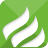线速度与角速度的关系是什么 二者有哪些区别

2021-12-30 17:51 来源： 高考学习网 本文影响了：494人匀速圆周运动的相关公式

1、v（线速度）=ΔS/Δt=2πr/T=ωr=2πrf(S代表弧长，t代表时间，r代表半径,f代表频率)

2、ω（角速度）=Δθ/Δt=2π/T=2πn（θ表示角度或者弧度）

3、T（周期）=2πr/v=2π/ω

4、n（转速）=1/T=v/2πr=ω/2π

5、Fn（向心力）=mrω^2=mv^2/r=mr4π^2/T^2=mr4π^2f^2

6、an（向心加速度）=rω^2=v^2/r=r4π^2/T^2=r4π^2n^2

7、vmin=√gr（过最高点时的条件）

8、fmin(过最高点时的对杆的压力)=mg-√gr（有杆支撑）

9、fmax(过最低点时的对杆的拉力)=mg+√gr（有杆）

0.509031s